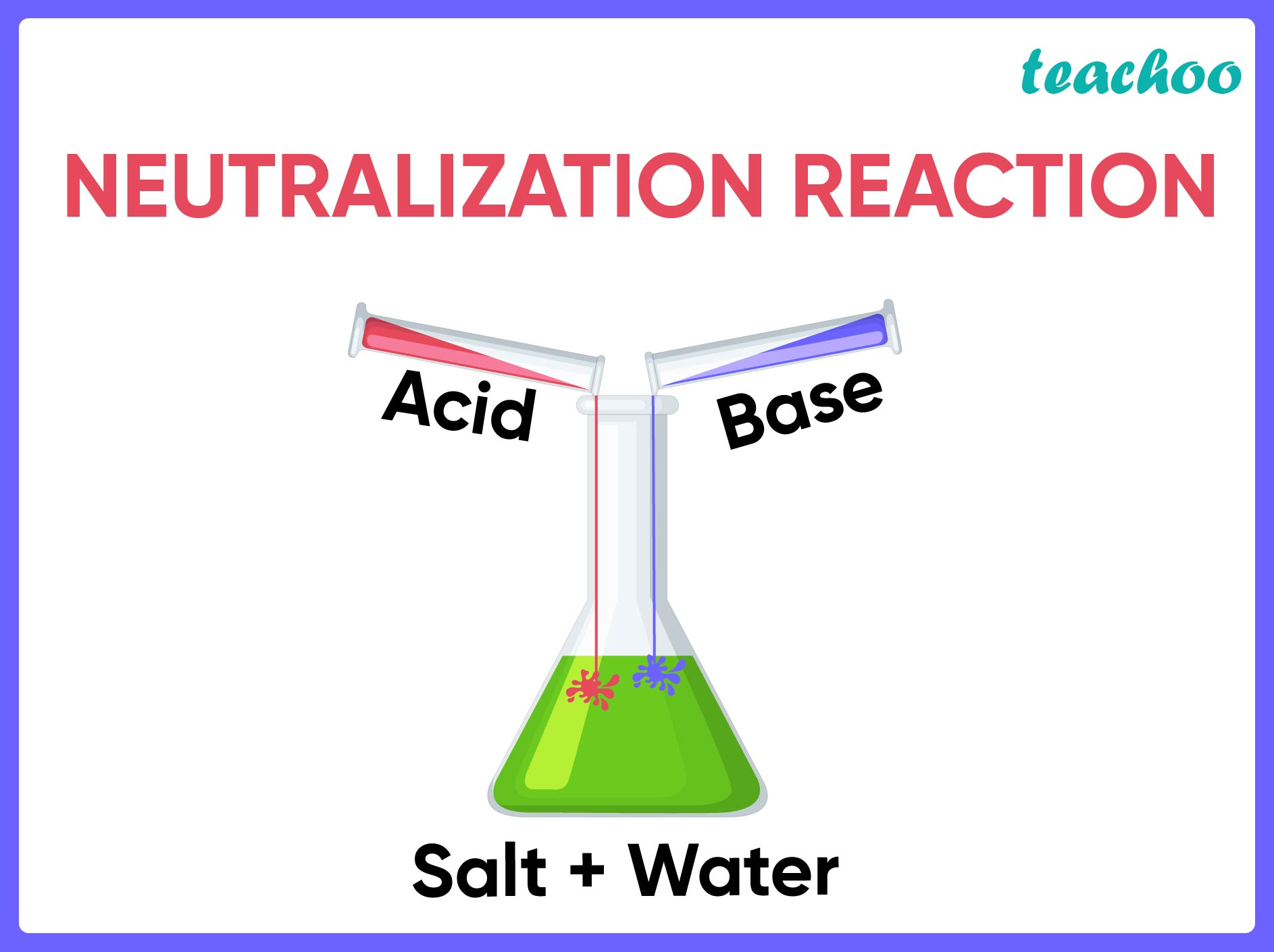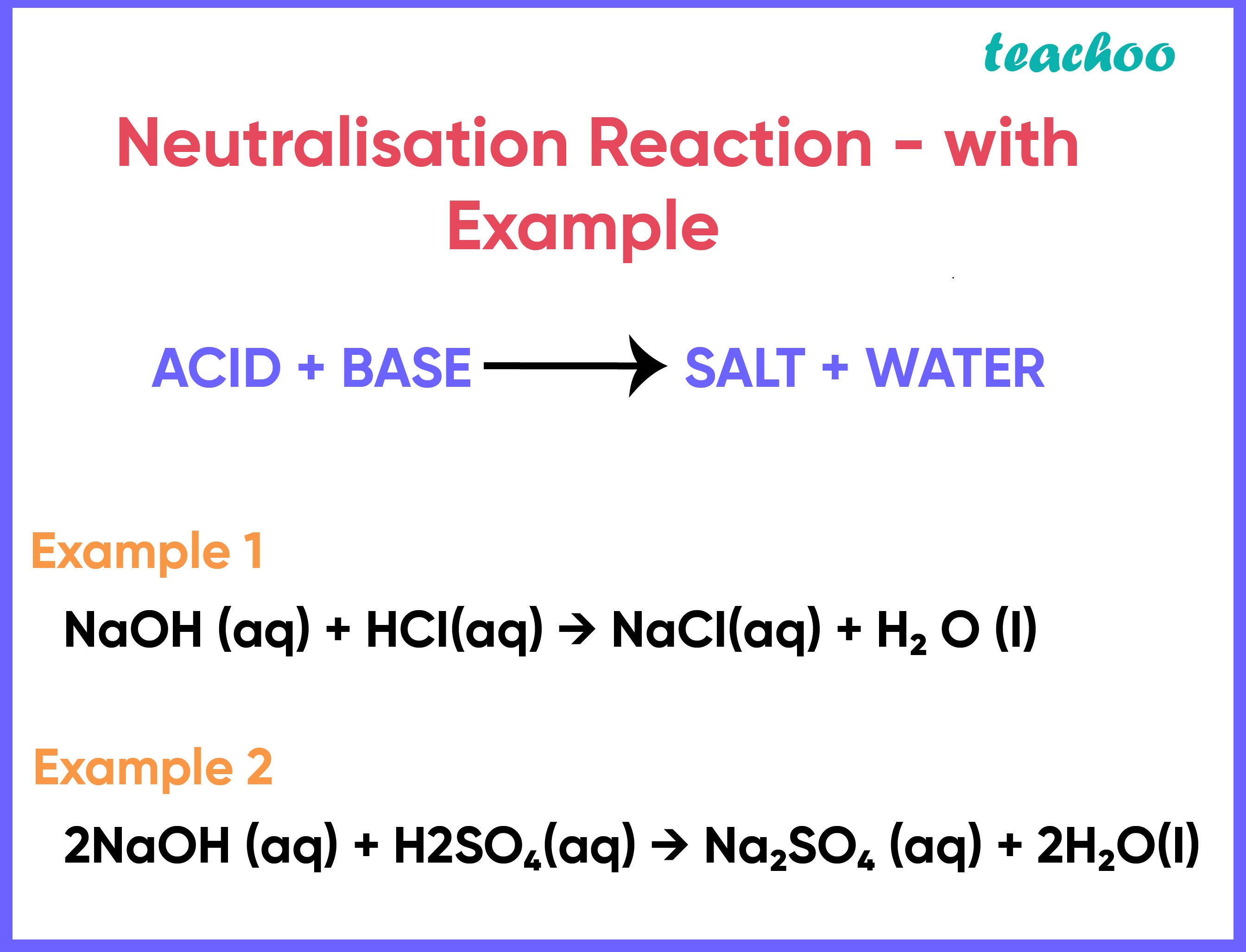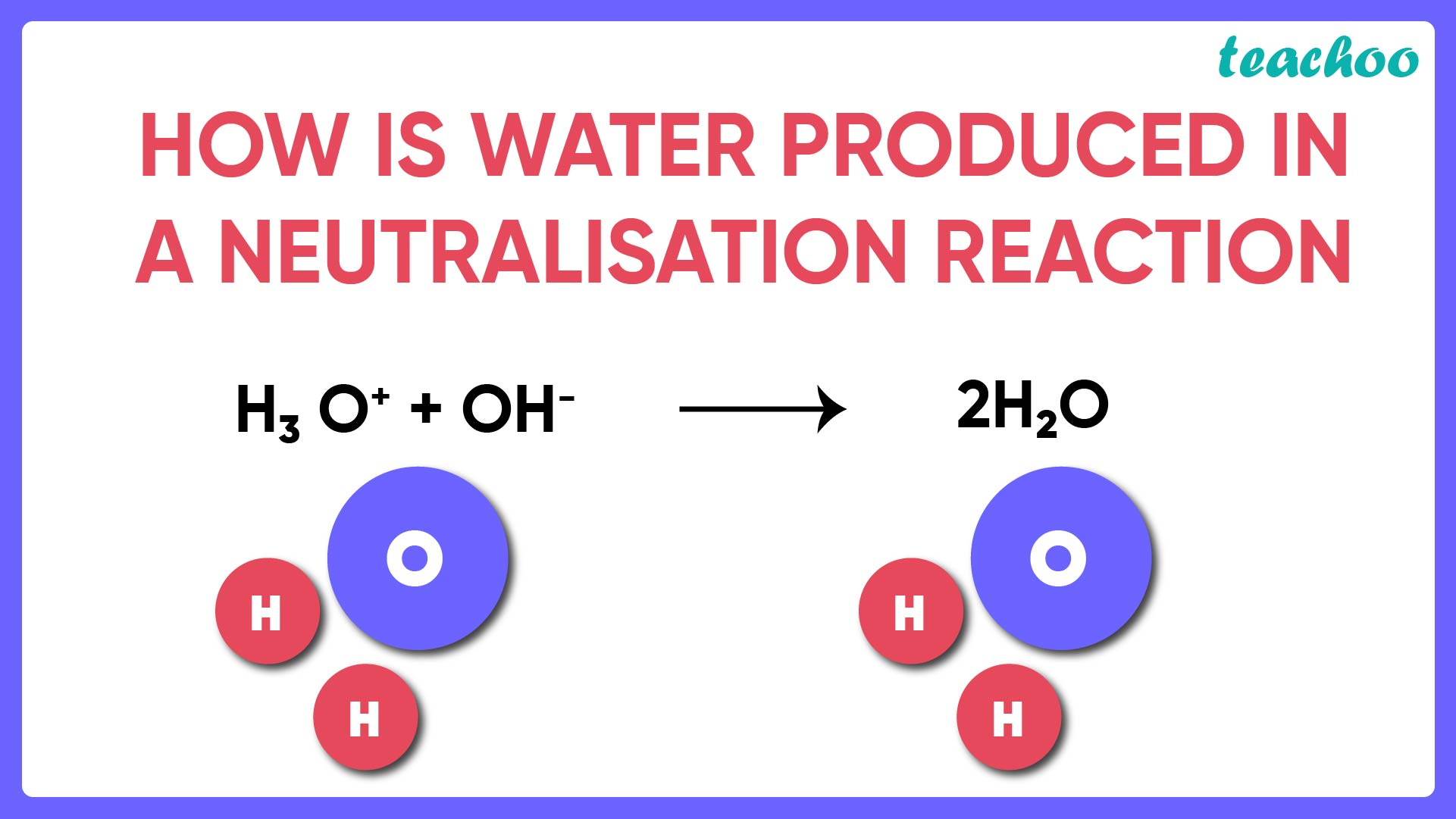Concepts

Class 10
Chapter 2 Class 10 - Acids, Bases and SaltsAcid reacting with Bases/ Alkalis (Neutralisation Reaction )

Acid + Base → Salt + Water

• When Sodium Hydroxide ( NaOH) , a base, reacts with Hydrochloric acid (HCl) , it forms the salt, Sodium Chloride (NaCl) and Water.

NaOH (aq) + HCl(aq) → NaCl (aq) + H 2 O (l)• When Sodium Hydroxide (NaOH) reacts with Sulphuric Acid (H 2 SO 4 ), Sodium sulphate (Na 2 SO 4 ) and Water (H 2 0)  are formed.

2NaOH (aq) + H 2 SO 4 (aq) → Na 2 SO 4 (aq) + 2H 2 O (l)

• In all these neutralisation reactions , the byproduct water is formed due to the combination of hydrogen ions present in the acid and hydroxide ions present in the base. The formation of water in an acid-base reaction occurs as follows:

H + (aq) + OH - (aq) → H 2 O (l)Learn in your speed, with individual attention - Teachoo Maths 1-on-1 Class